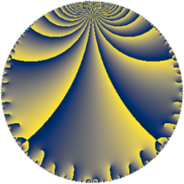# Properties

 Label 20.4.cLevel $20$ Weight $4$ Character orbit 20.c Rep. character $\chi_{20}(9,\cdot)$ Character field $\Q$ Dimension $2$ Newform subspaces $1$ Sturm bound $12$ Trace bound $0$

# Related objects

## Defining parameters

 Level: $$N$$ $$=$$ $$20 = 2^{2} \cdot 5$$ Weight: $$k$$ $$=$$ $$4$$ Character orbit: $$[\chi]$$ $$=$$ 20.c (of order $$2$$ and degree $$1$$) Character conductor: $$\operatorname{cond}(\chi)$$ $$=$$ $$5$$ Character field: $$\Q$$ Newform subspaces: $$1$$ Sturm bound: $$12$$ Trace bound: $$0$$

## Dimensions

The following table gives the dimensions of various subspaces of $$M_{4}(20, [\chi])$$.

Total New Old
Modular forms 12 2 10
Cusp forms 6 2 4
Eisenstein series 6 0 6

## Trace form

 $$2 q + 14 q^{5} - 98 q^{9} + O(q^{10})$$ $$2 q + 14 q^{5} - 98 q^{9} + 40 q^{11} + 152 q^{15} - 168 q^{19} + 152 q^{21} - 54 q^{25} + 12 q^{29} - 448 q^{31} - 152 q^{35} + 912 q^{39} + 532 q^{41} - 686 q^{45} + 534 q^{49} - 1216 q^{51} + 280 q^{55} - 56 q^{59} + 364 q^{61} - 912 q^{65} - 1064 q^{69} + 816 q^{71} + 2128 q^{75} + 96 q^{79} + 698 q^{81} + 1216 q^{85} - 3052 q^{89} - 912 q^{91} - 1176 q^{95} - 1960 q^{99} + O(q^{100})$$

## Decomposition of $$S_{4}^{\mathrm{new}}(20, [\chi])$$ into newform subspaces

Label Dim $A$ Field CM Traces $q$-expansion
$a_{2}$ $a_{3}$ $a_{5}$ $a_{7}$
20.4.c.a $2$ $1.180$ $$\Q(\sqrt{-19})$$ None $$0$$ $$0$$ $$14$$ $$0$$ $$q-\beta q^{3}+(7+\beta )q^{5}+\beta q^{7}-7^{2}q^{9}+\cdots$$

## Decomposition of $$S_{4}^{\mathrm{old}}(20, [\chi])$$ into lower level spaces

$$S_{4}^{\mathrm{old}}(20, [\chi]) \cong$$ $$S_{4}^{\mathrm{new}}(10, [\chi])$$$$^{\oplus 2}$$